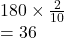## Angles of triangle are in the ratio of 23:5. What is the Size of the smallest angle?​

Question

Angles of triangle are in the ratio of 23:5. What is the Size of the smallest angle?​

in progress 0
7 months 2021-07-21T01:34:31+00:00 2 Answers 1 views 0

The smallest angle of a triangle is 36°.

Step-by-step explanation:

Given, the ratio of angles of a triangle is 2 : 3 : 5

Let the angles of a triangle be ∠A, ∠B and ∠C.

∠A = 2x, ∠B = 3x, ∠C = 5x

∠A+∠B + ∠C= 180°

[sum of all the angles of a triangle is 180°]

2x + 3x + 5x = 180°

10x = 180°

x=180°/10 =18°

∠A=2x=2 x 18° = 36°

∠B = 3x = 3 x 18° = 54°

∠C = 5x = 5 x 18° = 90°

Hence, the smallest angle of a triangle is 36°.

HOPE IT HELPS!!!